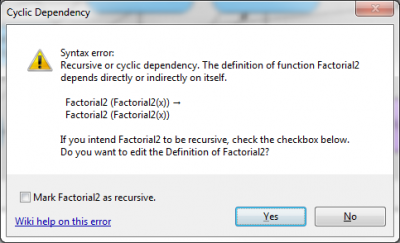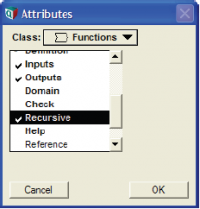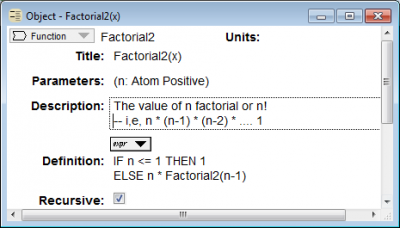# Recursion

A recursive function is a function that calls itself within its definition. Those new to recursion may find this a bit odd. Experienced programmers know that recursion sometimes the simplest way to define a function, and occasionally the only way. As an example, consider this definition of factorial:

Function Factorial2(n: Positive Atom)
Definition: IF n <= 1 THEN 1 ELSE n*Factorial2(n - 1)

If the parameter, n, is greater than 1, Factorial2 calls itself with the actual parameter value n - 1. Otherwise, it simply returns 1. Like any recursive function, it has a termination condition to tell it when to stop recursion — in this case, when n <= 1.

Tip
We named this function Factorial2 to distinguish it from the built-in function Factorial, which does the same.

### Enable a function to be recursive

When you first try to define a function that refers to itself, it complains about a cyclic dependency loop with an error message like this:The easiest way to enable recursion, is simply to click the checkbox in this error dialog.

Another way is to set the Recursive attribute explicitly. First you must display the Recursive for Functions:

1. Select Attributes... from the Object menu.2. Select Functions from the Class menu.
3. Scroll down the list of attributes and click Recursive twice, so that it shows √, meaning that the recursive attribute is displayed for each function in its Object window and the Attribute panel.
4. Check OK to close Attributes dialog.

Then, for each function for which you wish to enable recursion:

1. Open the Object window for the function by double-clicking its node (or select the node and click its Object button).
1. Click the checkbox in its Recursive attribute.### Recursive example for Prime Factors

As another example, consider this recursive function to compute a list of the prime factors of an integer, x, equal to or greater than y:

Function Prime_factors(x, y: Positive Atom)
Definition:
Var n := Floor(x/y);
IF n < y THEN [x]
ELSE IF x = n*y THEN Concat([y], Factors(n, y))
ELSE Prime_factors(x, y + 1)

Factors(60, 2) → [2, 2, 3, 5]

In essence, Prime_factors says to compute n as x divided by y, rounded down. If y is greater than n, then x is the last factor, so return x as a list. If x is an exact factor of y, then concatenate x with any factors of n, equal or greater than n. Otherwise, try y + 1 as a factor.

Tip
To prevent infinite recursion accidents, it stops and gives a warning if the stack reaches a depth of 256 function calls.